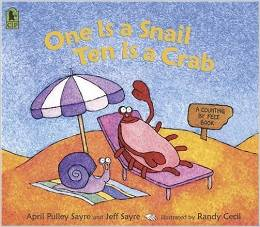Tens and Ones to 50

13 teachers like this lesson
Print Lesson

Objective

SWBAT group objects to show numbers to 50 as tens and ones.

Big Idea

Tens, Tens! In this lesson students will use base ten blocks to build numbers to 50.

Activating Strategy

10 minutes

In this lesson, children use base-ten blocks to represent tens and ones. Base-ten blocks are pregrouped models that are efficient to use, but children cannot take them apart or put them together to show number relationships.  Children may ask why they are using blocks instead of connecting cubes. I explain that with the blocks it is quicker to show greater numbers. Instead of connecting 10 cubes to make 1 ten, they can trade 10 ones for 1 ten.

I like to begin this lesson by reading, “One is a Snail, Ten is a Crab”.  I like this book because it is engaging for the students, and they love counting the feet on the animals.  The book uses various combinations of animals’ feet to count to 100.(A video of the read aloud can also be found here on You Tube.)

I then distribute connecting cubes to pairs of children and have children show 15 cubes.

• Predict how many tens you can make. How many ones will you have left over?

Partners should then work together to connect cubes to model 15 as 1 ten and 5 ones.

Teaching Strategies

15 minutes

I then display the Tens and Ones to 50.ppt, and read the following aloud:

Use 23 connecting cubes and show them as tens and ones. I then have a student come to the board and draw their model.

• What have you learned about making a ten that can help you show 23 as tens and ones?  (I know that 10 ones are equal to 1 ten. So I can make tens, and the leftover cubes are the ones.)

After children represent their work, allow them to share their pictures.

• How many tens did you make? How many ones are leftover? (I made 2 tens. I have 3 ones leftover.)

Some children may have made 1 ten with 13 ones leftover. Explain to children that this is a correct way to show the tens and ones in a two-digit number, but that it's not efficient because they can still can trade 10 more ones for 1 ten. Encourage them to make as many tens as possible.

Distribute tens and ones base-ten blocks. I then explain to children that these models can also be used to show tens and ones. Show children how to use base-ten blocks to model the number 23. As a class, discuss how this new model compares with the model that used connecting cubes.

Have children explore showing the numbers 18, 31, and 27 as tens and ones.

In this lesson, children represent tens and ones with paper and pencil for quick pictures and with concrete models in the form of base-ten blocks.  (MP5)  They begin to move from a concrete stage of representing numbers using connecting cubes, to modeling by drawing abstract pictures with quick drawings.

Independent Practice

30 minutes

Work through the model on the top of their Tens and Ones to 50_worksheet.docx with children (also found on the PPT). For 24, have children count the tens and trace the number below them. I then have children count the ones and trace the number. Repeat for the model of 42.

• What do the two digits in the number 24 stand for?  (The digit on the left is the number of tens. 24 has 2 tens. The digit on the right is the number of ones. 24 has 4 ones.)
• Is the value of the digit 2 the same in both 24 and 42? Explain. (No.  In 24, the 2 has a value of 2 tens, or 20. In 42, the 2 has a value of 2 ones, or 2.)
• How do you know that 42 has 4 tens?  (The 4 is on the left and the 2 is on the right, so 42 has 4 tens and 2 ones.)

I then allow students to continue to work independently on the rest of the worksheet.

Some students may reverse the digits when writing the numbers.  To help connect 3 tens 5 ones to 35, suggest that children work from left to right, recording the number of tens first, and then the number of ones.  To help students work from left to right so that they understand the place value of tens and ones, I encourage them to draw a T chart on their paper, labeling the sides "Tens" and "Ones"

Closing/Summarizing

5 minutes

To close out the lesson, I have students get their math journal.  I instruct the students to write a number from 20 to 50 that has both tens and ones. Use pictures and words to show the tens and ones.